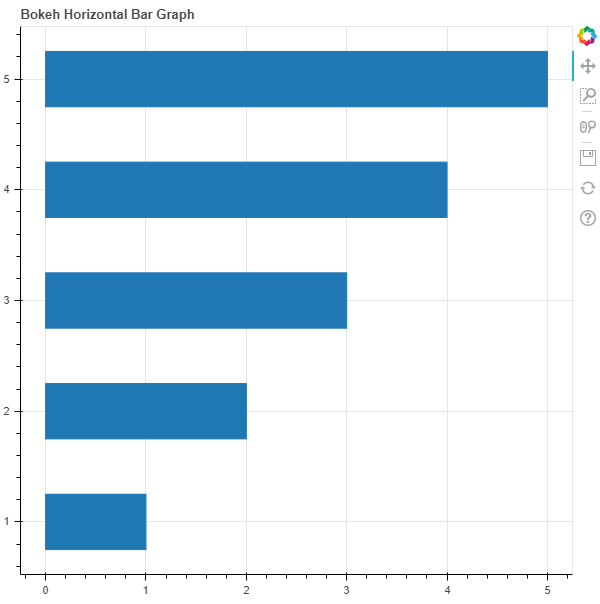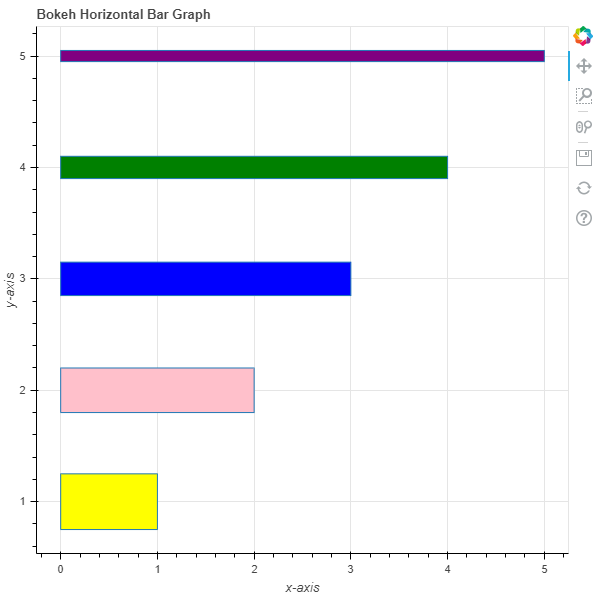Open in App
Not now

# Python Bokeh – Plotting Horizontal Bar Graphs

• Last Updated : 03 Jul, 2020

Bokeh is a Python interactive data visualization. It renders its plots using HTML and JavaScript. It targets modern web browsers for presentation providing elegant, concise construction of novel graphics with high-performance interactivity.

Bokeh can be used to plot horizontal bar graphs. Plotting horizontal bar graphs can be done using the `hbar()` method of the `plotting` module.

## plotting.figure.hbar()

Syntax : hbar(parameters)

Parameters :

• y : y-coordinates of the center of the horizontal bars
• height : thickness of the horizontal bars
• right : x-coordinates of the right edges
• left : x-coordinates of the left edges, default is 0
• fill_alpha : fill alpha value of the horizontal bars
• fill_color : fill color value of the horizontal bars
• hatch_alpha : hatch alpha value of the horizontal bars, default is 1
• hatch_color : hatch color value of the horizontal bars, default is black
• hatch_extra : hatch extra value of the horizontal bars
• hatch_pattern : hatch pattern value of the horizontal bars
• hatch_scale : hatch scale value of the horizontal bars, default is 12
• hatch_weight : hatch weight value of the horizontal bars, default is 1
• line_alpha : percentage value of line alpha, default is 1
• line_cap : value of line cap for the line, default is butt
• line_color : color of the line, default is black
• line_dash : value of line dash such as :
• solid
• dashed
• dotted
• dotdash
• dashdot

default is solid

• line_dash_offset : value of line dash offset, default is 0
• line_join : value of line join, default in bevel
• line_width : value of the width of the line, default is 1
• name : user-supplied name for the model
• tags : user-supplied values for the model

Other Parameters :

• alpha : sets all alpha keyword arguments at once
• color : sets all color keyword arguments at once
• legend_field : name of a column in the data source that should be used
• legend_group : name of a column in the data source that should be used
• legend_label : labels the legend entry
• muted : determines whether the glyph should be rendered as muted or not, default is False
• name : optional user-supplied name to attach to the renderer
• source : user-supplied data source
• view : view for filtering the data source
• visible : determines whether the glyph should be rendered or not, default is True
• x_range_name : name of an extra range to use for mapping x-coordinates
• y_range_name : name of an extra range to use for mapping y-coordinates
• level : specifies the render level order for this glyph

Returns : an object of class `GlyphRenderer`

Example 1 :In this example we will be using the default values for plotting the graph.

 `# importing the modules ` `from` `bokeh.plotting ``import` `figure, output_file, show ` ` `  `# file to save the model ` `output_file(``"gfg.html"``) ` `     `  `# instantiating the figure object ` `graph ``=` `figure(title ``=` `"Bokeh Horizontal Bar Graph"``) ` ` `  `# y-coordinates to be plotted ` `y ``=` `[``1``, ``2``, ``3``, ``4``, ``5``] ` ` `  `# x-coordinates of the right edges ` `right ``=` `[``1``, ``2``, ``3``, ``4``, ``5``] ` ` `  `# height / thickness of the bars  ` `height ``=` `0.5` ` `  `# plotting the graph ` `graph.hbar(y, ` `           ``right ``=` `right, ` `           ``height ``=` `height) ` ` `  `# displaying the model ` `show(graph) `

Output :Example 2 :In this example we will be plotting horizontal bars with different parameters.

 `# importing the modules ` `from` `bokeh.plotting ``import` `figure, output_file, show ` ` `  `# file to save the model ` `output_file(``"gfg.html"``) ` `     `  `# instantiating the figure object ` `graph ``=` `figure(title ``=` `"Bokeh Horizontal Bar Graph"``) ` ` `  `# name of the x-axis ` `graph.xaxis.axis_label ``=` `"x-axis"` `     `  `# name of the y-axis ` `graph.yaxis.axis_label ``=` `"y-axis"` ` `  `# y-coordinates to be plotted ` `y ``=` `[``1``, ``2``, ``3``, ``4``, ``5``] ` ` `  `# x-coordinates of the right edges ` `right ``=` `[``1``, ``2``, ``3``, ``4``, ``5``] ` ` `  `# height / thickness of the bars  ` `height ``=` `[``0.5``, ``0.4``, ``0.3``, ``0.2``, ``0.1``] ` ` `  `# color values of the bars ` `fill_color ``=` `[``"yellow"``, ``"pink"``, ``"blue"``, ``"green"``, ``"purple"``] ` ` `  `# plotting the graph ` `graph.hbar(y, ` `           ``right ``=` `right, ` `           ``height ``=` `height, ` `           ``fill_color ``=` `fill_color) ` ` `  `# displaying the model ` `show(graph) `

Output :My Personal Notes arrow_drop_up
Related Articles